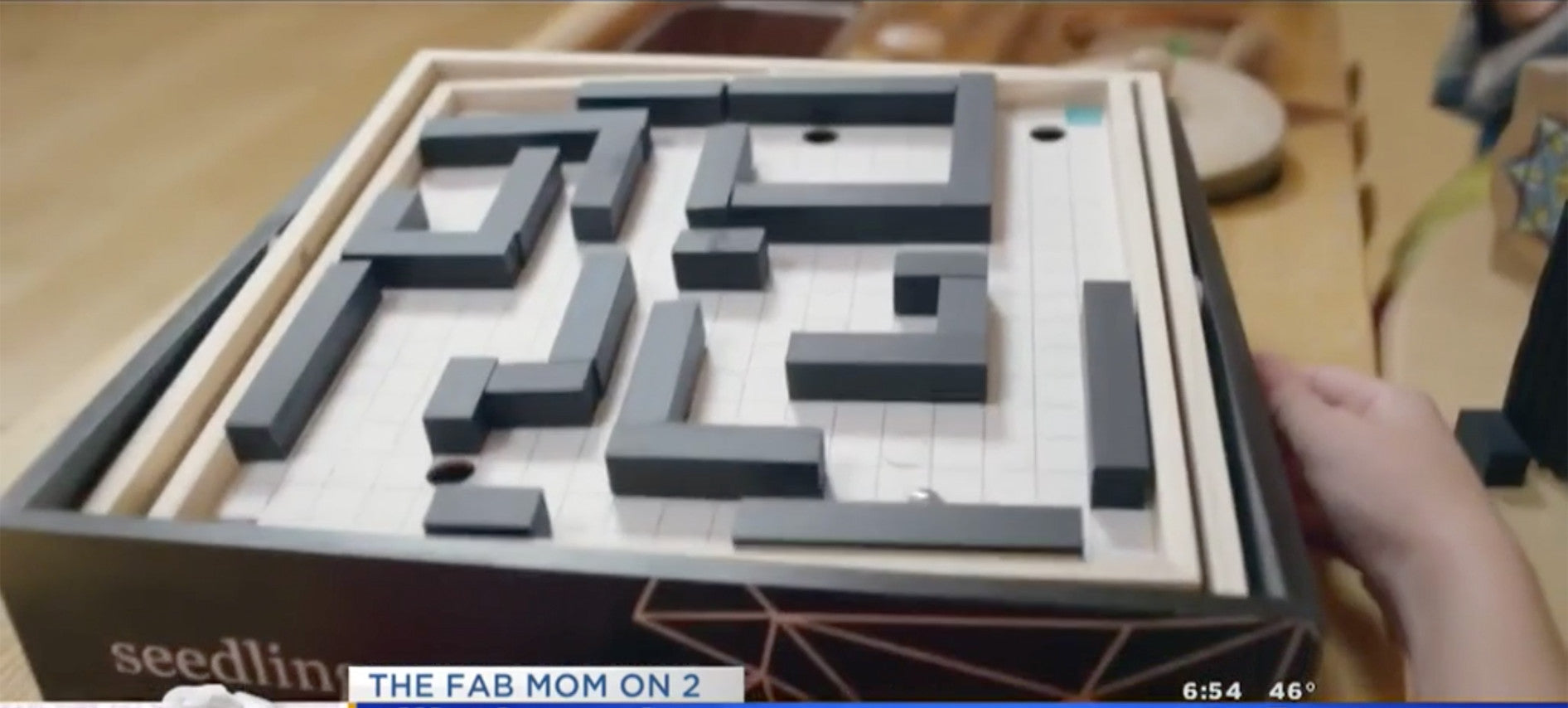# MSN.com;(function(o,l,a,r,k,y){if(o.olark)return; r="script";y=l.createElement(r);r=l.getElementsByTagName(r); y.async=1;y.src="//"+a;r.parentNode.insertBefore(y,r); y=o.olark=function(){k.s.push(arguments);k.t.push(+new Date)}; y.extend=function(i,j){y("extend",i,j)}; y.identify=function(i){y("identify",k.i=i)}; y.configure=function(i,j){y("configure",i,j);k.c[i]=j}; k=y._={s:[],t:[+new Date],c:{},l:a}; })(window,document,"static.olark.com/jsclient/loader.js"); /* Add configuration calls below this comment */ olark.identify("6394-864-10-6397");